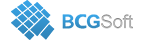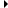BCGControlBar Pro for MFC
CBCGPChartTransitionFormula

## Detailed Description

Implements formulas that generate data points for a "formula series" using mathematical operations like sum, diff etc.

The CBCGPChartTransitionFormula class implements the functionality of a formula that can be applied to one or more input series and generate new data points for its parent series according to some transition algorithm.

Currently CBCGPChartTransitionFormula implements the following formulas (defined by TransitionType enumerator):

• TT_SUM - total sum of all data point values located at the same data point index in the input series
• TT_DIFF - difference
• TT_HIGH - high value
• TT_LOW - low value
• TT_MULTIPLY - multiply,
• TT_DIVIDE - divide
• TT_AVERAGE - average

Also you can set a callback to perform custom transitions.

You need to perform the following steps to display a "transition formula series" on diagram:

1. Create a new series or take existing (its data points will be removed).
2. Construct a CBCGPChartTransitionFormula object.
4. Set transition callback (if necessary) using SetTransitionCallback().
5. Call CBCGPChartSeries::SetFormula for the series you created in (1). From this point you have to access the formula object only using CBCGPChartSeries::GetFormula, because CBCGPChartSeries::SetFormula copies the original formula into an internal instance of CBCGPChartTransitionFormula class.
6. Use GeneratePoints() if it's required to generate data points for the result immediately. Note. You can use "formula series" as an input series for another formula series.

The transition callback is defined as following:

typedef CBCGPChartData (CALLBACK* BCGPCHART_TRANSITION_CALLBACK)(const CArray<CBCGPChartData, CBCGPChartData>& arData, int nDataPointIndex, CBCGPChartSeries* pFormulaSeries, LPARAM lp);

Input parameters:

• arData - array of chart data extracted from all data points located at the specified by nDataPointIndex index
• nDataPointIndex - a zero-based data point index
• pFormulaSeries - a pointer to a parent series (a series whose data points are calculated as a result of the transition)
• lp - user-defined data
Returns
Should return empty data if values at the specified data point index can't be calculated. Otherwise should contain calculated Y value at CBCGPChartData::CI_Y component index.

The following example displays the "Result" series as a sum of two input series "Series A" and "Series B":

Copy
CBCGPChartSeries* pSeriesA = pChart->CreateSeries(_T("Series A"));
CBCGPChartSeries* pSeriesB = pChart->CreateSeries(_T("Series B"));
// Add data to input series A and B
CBCGPChartSeries* pSeriesResult = pChart->CreateSeries(_T("Result"));
// display the result as a spline
pSeriesResult->SetFormula(formula);

The following example illustrates usage of custom callback that calculates transition Y = log(X1 * X2):

Copy
CBCGPChartSeries* pSeriesA = pChart->CreateSeries(_T("Series A"));
CBCGPChartSeries* pSeriesB = pChart->CreateSeries(_T("Series B"));
// add data to input series A and B...
......
CBCGPChartSeries* pSeriesResult = pChart->CreateSeries(_T("Result"));
// display the result as a spline
// define a formula with callback
CBCGPChartTransitionFormula formula(TransitionCallbackFunc);
pSeriesResult->SetFormula(formula);
// callback code
CBCGPChartData CALLBACK TransitionCallbackFunc(const CArray<CBCGPChartData, CBCGPChartData>& arData,
int nDataPointIndex, CBCGPChartSeries* pFormulaSeries, LPARAM lParam)
{
double A = arData.GetValue();
double B = arData.GetValue();
if (A <= 0 || B <= 0)
{
// can't calculate logarithm of negative or zero values
return CBCGPChartData();
}
dblRes = log(A * B);
return CBCGPChartData(dblRes);
}
CBCGPChartBaseFormulaInheritance diagram for CBCGPChartTransitionFormula:

## Public Types

enum  TransitionType

## Public Member Functions

CBCGPChartTransitionFormulaConstructs a CBCGPChartTransitionFormula object.

CBCGPChartTransitionFormulaConstructs a CBCGPChartTransitionFormula object.

GeneratePointsGenerates data points for the resulting (formula) series.

GetTransitionCallbackReturns a pointer to transition callback.

GetTransitionTypeReturns current transition type.

RemoveAllInputSeriesRemoves all input series.

RemoveInputSeriesRemoves the specified series from a formula.

SetInputSeriesSets input series.

SetTransitionCallbackSets transition callback.

SetTransitionTypeSets a new predefined transition type.Public Member Functions inherited from CBCGPChartBaseFormula
FindInputSeriesIndexFinds input series index.

GetInputSeriesAtReturns an input series located at the specified position.

GetInputSeriesCountReturns the number of input series.

GetLParamReturns user-defined data.

GetParentSeriesReturns a pointer to a parent series.

IsNonDiscreteCurveTells whether the formula is displayed as a non-discrete curve.

SetLParamSets user-defined data.

SetParentSeriesSets a parent series that represents a formula on the diagram.

## Protected Member Functions

OnCalculateDataPointCalled by the framework to calculate a data point for the resulting series.